Email us to get an instant 20% discount on highly effective K-12 Math & English kwizNET Programs!

#### Online Quiz (WorksheetABCD)

Questions Per Quiz = 2 4 6 8 10

### Grade 4 - Mathematics8.9 Types of Fractions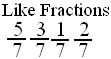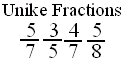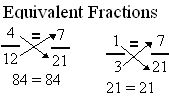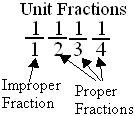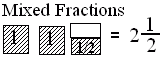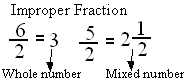Directions: Answer the following questions. Also write at least 5 examples of each type of fractions.
 Q 1: When a products obtained by cross multiplication give the same number, then the fractions are ____(or equal).propermixedequivalent Q 2: Fraction with numerator 1 is called a unit fraction. Are all unit fractions proper?NoYes Q 3: A number with a whole number and a proper fraction is amixed numberproper number Q 4: A mixed number denotes the sum of a whole number-part and proper fraction part.TrueFalse Question 5: This question is available to subscribers only! Question 6: This question is available to subscribers only!